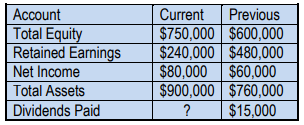Clutch Prep is now a part of Pearson
Ch. 14 - Financial Statement AnalysisWorksheetSee all chapters

# Ratios: Payout Ratio

See all sections
Sections
Horizontal Analysis
Vertical Analysis
Common-sized Statements
Trend Percentages
Discontinued Operations and Extraordinary Items
Introduction to Ratios
Ratios: Earnings Per Share (EPS)
Ratios: Working Capital and the Current Ratio
Ratios: Quick (Acid Test) Ratio
Ratios: Gross Profit Rate
Ratios: Profit Margin
Ratios: Quality of Earnings Ratio
Ratios: Inventory Turnover
Ratios: Average Days in Inventory
Ratios: Accounts Receivable (AR) Turnover
Ratios: Average Collection Period (Days Sales Outstanding)
Ratios: Return on Assets (ROA)
Ratios: Total Asset Turnover
Ratios: Fixed Asset Turnover
Ratios: Profit Margin x Asset Turnover = Return On Assets
Ratios: Accounts Payable Turnover
Ratios: Days Payable Outstanding (DPO)
Ratios: Times Interest Earned (TIE)
Ratios: Debt to Asset Ratio
Ratios: Debt to Equity Ratio
Ratios: Payout Ratio
Ratios: Dividend Yield Ratio
Ratios: Return on Equity (ROE)
Ratios: DuPont Model for Return on Equity (ROE)
Ratios: Free Cash Flow
Ratios: Price-Earnings Ratio (PE Ratio)
Ratios: Book Value per Share of Common Stock
Ratios: Cash to Monthly Cash Expenses
Ratios: Cash Return on Assets
Ratios: Economic Return from Investing
Ratios: Capital Acquisition Ratio

Concept #1: Ratios: Payout Ratio

Practice: ConsistoCo has a policy to maintain a constant payout ratio from year to year. During the previous fiscal year, net income totaled $1,200,000 and ConsistoCo paid$240,000 in dividends. This year, due to the settlement of a lawsuit, the company had net income of \$700,000. What amount of dividends would investors expect ConsistoCo to declare this year?

Practice: Dive Company maintains a policy to have a consistent payout ratio from year to year. Selected financial information for the company is as follows:What amount of dividends would the company pay during the current year?